MORE IN Mechanics of Solids
GTU Civil Engineering (Semester 3)
Mechanics of Solids
December 2014
Total marks: --
Total time: --
INSTRUCTIONS
(1) Assume appropriate data and state your reasons
(2) Marks are given to the right of every question
(3) Draw neat diagrams wherever necessary

1 (a) Enumerate various types of supports with neat symbolic sketches, showing possible reactions.
3 M
1 (b) Define (i) Poisson's ratio (ii) Volumetric Strain (iii) Modulus of rigidity (iv) Bulk modulus.
4 M
1 (c) A cast iron water pipe of 500 mm inside diameter and 20 mm thick, is supported over a span of 10 meters. Find the maximum bending stress in the pipe metal, when the pipe is running full. Take density of cast iron = 70.6 kN/m3 and water = 9.8 kN/m3.
7 M

2 (a) State "Law of parallelogram of forces". Determine magnitude and direction of the resultant of the two forces shown in fig. (i)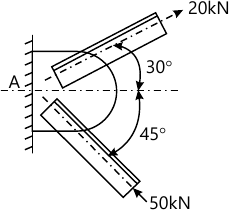7 M
Answer any one question from Q2 (b) & Q2 (c)
2 (b) State Pappus - Guldinus theorems. Using these theorems derive the formula for surface area (A) and volume (V) for sphere of radius 'r'.
7 M
2 (c) Derive relation between the rate of loading, shear force and bending moment with usual notations.
7 M

Answer any two question from Q3 (a), (b) & Q3 (c), (d)
3 (a) A stepped circular bar ABCD is axially loaded as shown in fig. (ii) is in equilibrium. Find unknown force P, and calculate stresses in each part and total change in length of the bar. Take Ecopper = 100 GPa, Ebrass = 80 GPa and Esteel = 200GPa.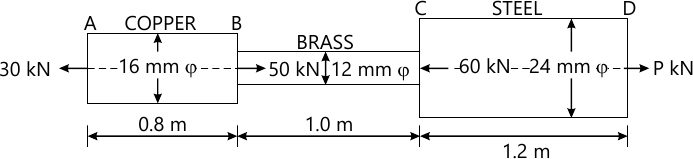7 M
3 (b) For an element shown in fig. (iii), find (i) principal stresses and location of corresponding principal planes (ii) Maximum shear stress and location of planes containing it.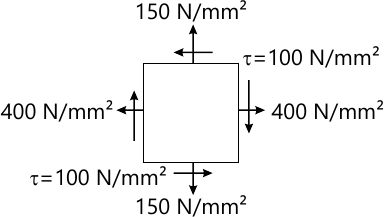7 M
3 (c) Determine the resultant of the forces 100 N, 200 N, 300 N, 400 N and 500 N are acting on one of the vertex of a regular hexagon, towards the other vertices, taken in order as shown in fig. (iv).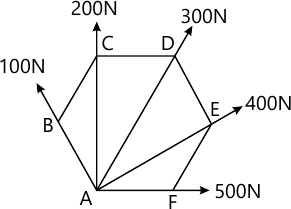7 M
3 (d) A ladder AB is 4.5 m long and 450 N weight, rests on a rough horizontal floor at end B and vertical wall at A, making 60° with horizontal. The coefficient of static friction is 0.4 for all contact surfaces. A man of 800 N weight climbs on the ladder. Determine the minimum distance travelled on the ladder, when it is on the verge of slipping.
7 M

Answer any two question from Q4 (a), (b) & Q4 (c), (b)
4 (a) A beam is loaded as shown in fig. (v). Calculate shear force and bending moment at points A, B, C, D and E and draw shear force and bending moment diagrams. Also find point of contraflexure.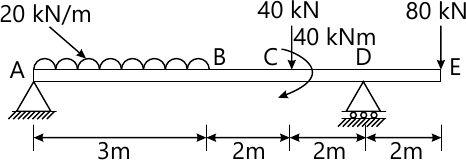7 M
4 (b) A short concrete column 450mm × 450mm in section is axially loaded to 500 kN. The column is strengthened by four, 16mm diameter steel bars each one at corner. Calculate stresses in concrete and steel. Take Ec = 14 GPa and Es = 210 Gpa.
7 M
4 (c) A T - shaped cross section of a beam has flange = 200 × 50 mm and web = 50×200 mm in size, is subjected to a vertical shear force of 100 kN. Calculate the shear stresses at important points and draw shear stress distribution diagram. Take moment of inertia about horizontal neutral axis = 113.4 × 106 mm4.
7 M
4 (d) Determine moment of inertia about horizontal centroidal axis for the section shown in fig. (vi)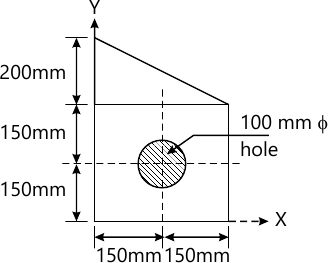7 M

Answer any two question from Q5(a), (b), (c) & Q5 (d), (e), (f)
5 (a) State conditions of equilibrium for coplanar and concurrent forces.
3 M
5 (b) State and explain Varignon's theorem, using illustrative example.
4 M
5 (c) State and explain Varignon's theorem, using illustrative example. In an assembly of steel rod of 20 mm diameter passes centrally through a copper tube 40 mm external diameter and 30 mm internal diameter. The tube is closed at both ends by rigid plates of negligible thickness, is initially stress free. If the temperature of the assembly is raised by 60° C, calculate stresses developed in copper and steel. Take Modulus of elasticity E for steel = 200 MPa and for copper= 100 MPa, Co efficient of thermal expansion α for steel = 12 x 10-6/° C and for copper = 18 x 10-6/°C.
4 M
5 (d) State laws of dry friction.
3 M
5 (e) Define couple and discuss its characteristics.
4 M
5 (f) A solid steel circular shaft is required to transmit a torque of 6.5 kNm. Determine minimum diameter of the shaft, if shear stress is limited to 40 N/mm2 and angle of twist should not exceed 0.5° per meter. Take Modulus of rigidity C=85 Gpa.
7 M

More question papers from Mechanics of Solids